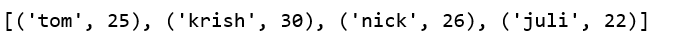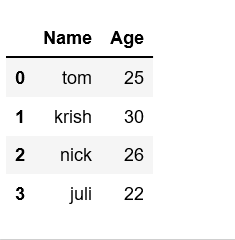Related Articles

# Create pandas dataframe from lists using zip

• Last Updated : 13 Nov, 2018

One of the way to create Pandas DataFrame is by using zip() function.

You can use the lists to create lists of tuples and create a dictionary from it. Then, this dictionary can be used to construct a dataframe.

zip() function creates the objects and that can be used to produce single item at a time. This function can create pandans DataFrames by merging two lists.

Suppose there are two lists of student data, first list holds the name of student and second list holds the age of student. Then we can have,

 `# List1``Name ``=` `[``'tom'``, ``'krish'``, ``'nick'``, ``'juli'``]`` ` `# List2``Age ``=` `[``25``, ``30``, ``26``, ``22``]`

Above two lists can be merged by using `list(zip())` function. Now, create the pandas DataFrame by calling `pd.DataFrame()` function.

 `# Python program to demonstrate creating``# pandas Datadaframe from lists using zip.`` ` `import` `pandas as pd`` ` `# List1``Name ``=` `[``'tom'``, ``'krish'``, ``'nick'``, ``'juli'``]`` ` `# List2``Age ``=` `[``25``, ``30``, ``26``, ``22``]`` ` `# get the list of tuples from two lists.``# and merge them by using zip().``list_of_tuples ``=` `list``(``zip``(Name, Age))`` ` `# Assign data to tuples.``list_of_tuples `

Output:`# Converting lists of tuples into``# pandas Dataframe.``df ``=` `pd.DataFrame(list_of_tuples, columns ``=` `[``'Name'``, ``'Age'``])``  ` `# Print data.``df`

Output:Attention geek! Strengthen your foundations with the Python Programming Foundation Course and learn the basics.

To begin with, your interview preparations Enhance your Data Structures concepts with the Python DS Course. And to begin with your Machine Learning Journey, join the Machine Learning – Basic Level Course

My Personal Notes arrow_drop_up